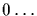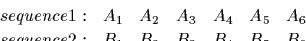Next: Point Accepted Mutations Up: Point Accepted Mutations and Previous: Point Accepted Mutations and

# Modeling Evolution

A mutation matrix, denoted by M, describes the probabilities of amino acid mutations for a given period of evolution.

The value 1-Mii then represents the probability of mutating away from i.

The matrix M corresponds to a model of evolution in which amino acids mutate randomly and independently from one another but according to some predefined probabilities. This is a Markovian model and, while simple, it is one of the best methods available for modeling evolution.

There are two main assumptions implicit in such a model: (1) amino acid substitutions subsequent in time are independent of preceding substitutions and (2) substitutions at specific positions in the protein sequence are independent of substitutions elsewhere in the sequence. Intrinisic properities of amino acids, like hydrophobicity, size, charge, etc. can be modelled by appropriate mutation matrices. However, we can not model dependencies which relate one amino acid characteristic to the characteristics of its neighbours. Of course, these assumption are not strictly true and readers are referred to  where the authors perform an analysis of the magnitude of this disparity.

Amino acids appear in nature with different frequencies. These frequencies are denoted by fi and correspond to the steady state of the Markov process defined by the matrix M, that is, the vector f is any of the columns ofor the eigenvector of Mwhose corresponding eigenvalue is 1 ().

We can not distinguish between a mutation from i into j and a mutation from j into i. This implies a simple relation for the entries of M:Next: Point Accepted Mutations Up: Point Accepted Mutations and Previous: Point Accepted Mutations and
Gaston Gonnet
1998-09-15